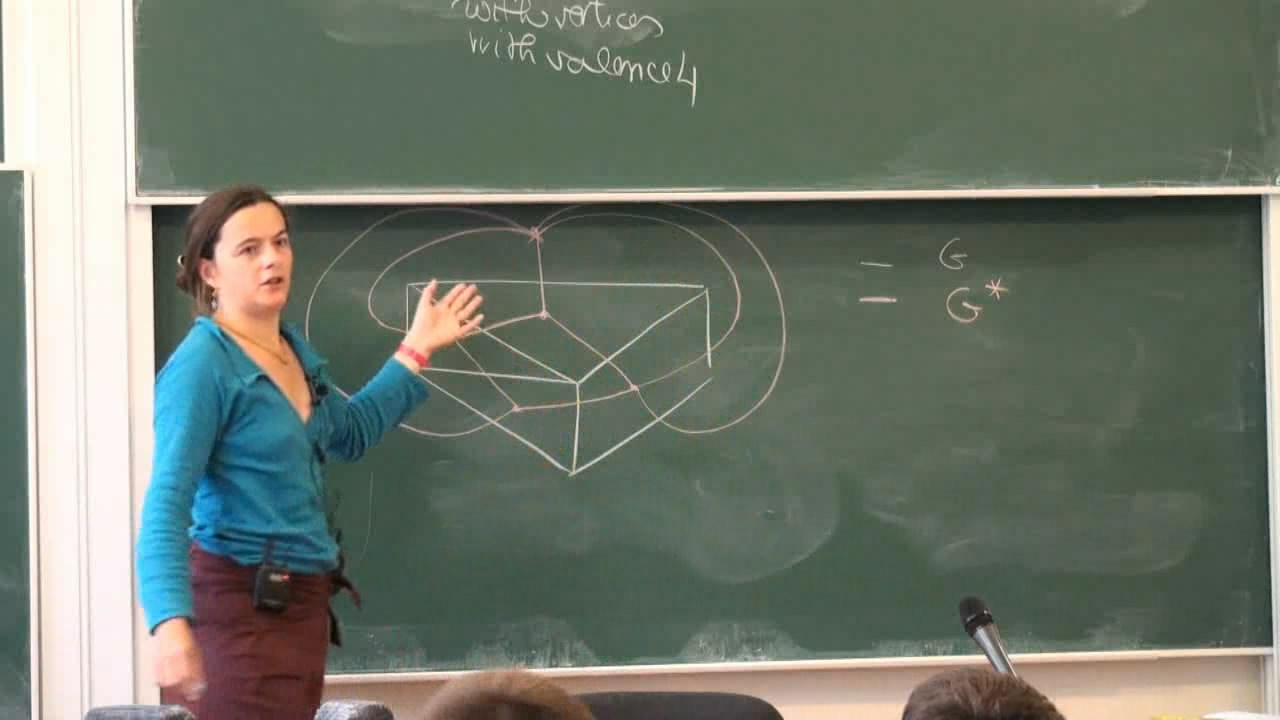# Random matrices and the Potts model on random graphs

Курс Хит
Предмет:
Лектор:Matrix integrals have long been used in physics to study properties of statistical models on random graphs. In this mini-course, we will analyze this relation in the case of the particular model of the Potts model on random planar maps. Planar maps are graphs which can be properly embedded into the sphere. The Potts model on a given graph is a model of random Q-coloring of the set of vertices with a build-in interaction such that two neighboring vertices prefer to have the same color. The underlying graph will be chosen randomly in the set of planar maps.

We will show that such a model is equivalent to some loop model on random graphs, where the random loops can be thought as the random boundary of regions of equal color. We will prove that such loop models can be represented by certain matrix integrals that can be easier to analyze (and compute) than the initial model itself. All the above notions will be introduced along the course and no prerequisite will be needed.

Курс прочитан в рамках St. Petersburg School in Probability and Statistical Physics.

Источник информации

3